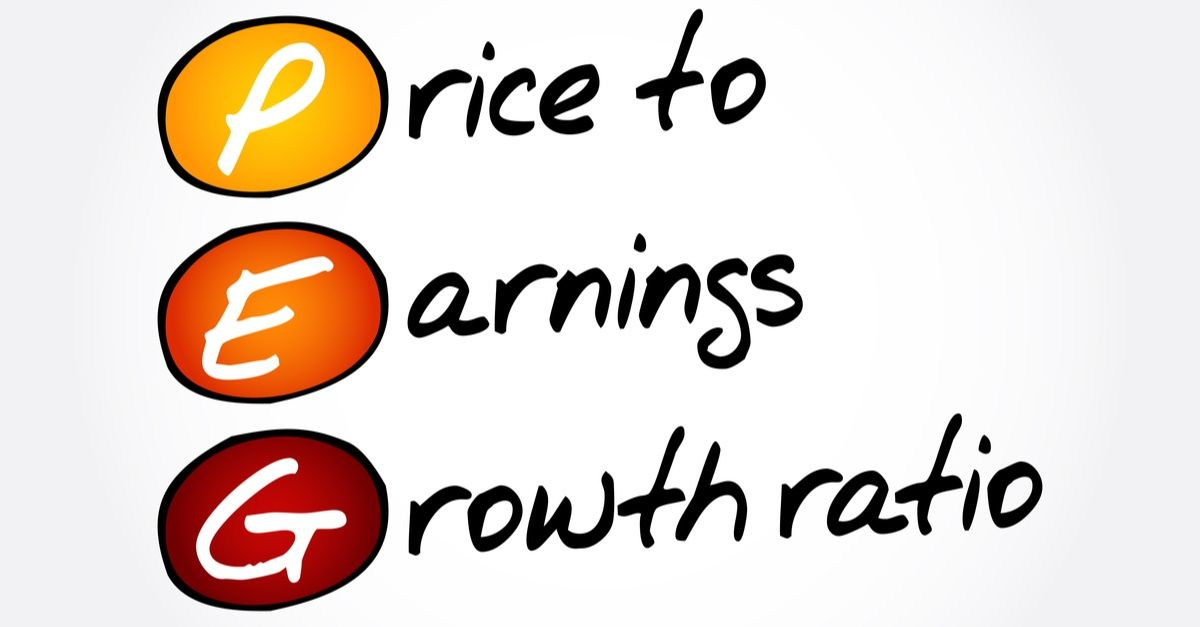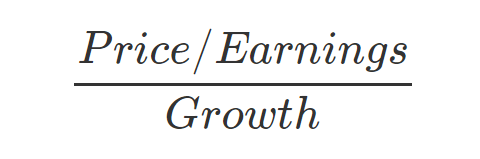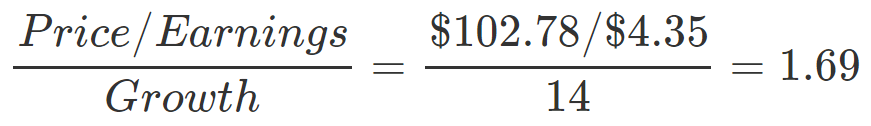# PEG Ratio: The Price/Earnings to Growth Ratio Explained

By Kris Gunnars, BSc | Feb 4, 2019The PEG ratio (price/earnings to growth) is a useful stock valuation measure. It is calculated by dividing a stock's price-to-earnings (PE) ratio with the company's earnings growth.

If you are trying to determine if a company's stock is expensive, cheap or fairly valued, then this is one of the best ratios to look at, especially for companies that are growing.

Instead of just looking at price-to-earnings, this ratio also factors in the growth rate of the company, which is crucial in understanding if something is reasonably valued or not.

Put simply, you take the price/earnings ratio and divide it by the earnings growth. Here is the formula:## How to use the PEG ratio formula to value a stock

To explain how this works, let's examine Microsoft's PEG ratio.

At the time of this writing, the stock price is \$102.78, while its earnings per share (EPS) in the last 12 months is \$4.35. If we divide the stock price with the earnings per share number, we see that Microsoft has a PE ratio of 23.62.

It is really impossible to tell just from looking at the PE ratio whether Microsoft stock is fairly valued or not. If they are not growing at all, then 23 is a very high PE ratio. But if they are growing fast and have great future prospects, then this PE ratio may be considered low.

To calculate the much more useful PEG ratio, we simply divide the PE ratio by the company's earnings growth rate.

By using Microsoft's EPS growth rate in the last quarter, which was about 14%, we find the PEG ratio in this way:So, Microsoft's PEG ratio is 1.69.

On the other hand, Facebook's PE ratio is around 22, while its earnings growth rate last year was 40%. This gives it a PEG ratio of 22/40 = 0.55.

Based on the PEG ratio alone, this would imply that Microsoft (MSFT) stock is a little expensive, while Facebook (META) stock is undervalued.

## What is a good PEG ratio?

The PEG ratio was popularized by legendary fund manager Peter Lynch.

According to him, a PEG ratio of 1 means that a stock is reasonably priced. This means that the company is fairly priced relative to its growth rate.

But a stock that is "much higher" than 1 is likely overvalued, while a stock that is "much lower" than 1 is likely undervalued.

To summarize:

• PEG over 1: overvalued (bad)
• PEG around 1: fairly valued (good)
• PEG under 1: undervalued (very good)

Keep in mind that 1 is not a precise cutoff. A stock could still be fairly valued or even cheap if its PEG ratio is 1.1 or 1.2, for example. The number 1 is just a useful ballpark figure for a quick evaluation.

## Which numbers to use in the formula?

Even though the PEG ratio may seem simple, it gets more complicated when you consider that there are different ways to calculate it. For example, there are different PE ratios and earnings growth rates to look at.

### Different PE ratios:

• PE ratio: uses the earnings per share (EPS) in the last 12 months
• Forward PE: uses the estimated earnings per share for the next year
• Adjusted PE: using adjusted EPS numbers to find the ratio

### Different growth rates:

• EPS growth last quarter
• EPS growth last year
• Estimated EPS growth the next 3 or 5 years
• Revenue growth last quarter or year

So depending on which numbers you use, the PEG ratio could be widely different. You need to look at the numbers for the company in context to decide which numbers are reasonable to use.

For example, if the PE ratio of a stock is either way too high or way too low due to recent one-time accounting events, then you shouldn't use this number to calculate the PEG ratio.

Instead, you can use the forward PE ratio, which uses the estimated earnings per share for the next year.

For the growth rate, you can either use the earnings growth rate or the revenue growth rate.

I personally prefer using the recent revenue growth rate because I think it is more indicative of the company's growth than earnings growth, which can vary widely based on one-time factors and accounting shenanigans.

For example, dividing the stock's forward PE by last quarter's revenue growth rate is a good place to start.

## Limitations of the PEG ratio

There is no one magic formula in investing.

Even though the PEG ratio is more useful than most others, it is still just a very simple mathematical formula and can not be used on its own to determine whether an investment is good or bad.

Investing is not just about numbers. You also need to look at qualitative factors like the company's management and competitive advantages.

In addition, it is not possible to use the PEG ratio for companies that don't turn a profit, because in this case their PE ratio is negative and doesn't work in the formula.

For these companies, other valuation ratios like price to sales (PS) or enterprise value / EBIT could be more useful.

## Conclusion

The PEG ratio is one of the most useful ways to quickly evaluate the stock price of a company that is still growing.

If you can find a stock whose price to earnings ratio is lower than its revenue or earnings growth rate (PEG under 1), then you are likely to have an undervalued investment on your hands.